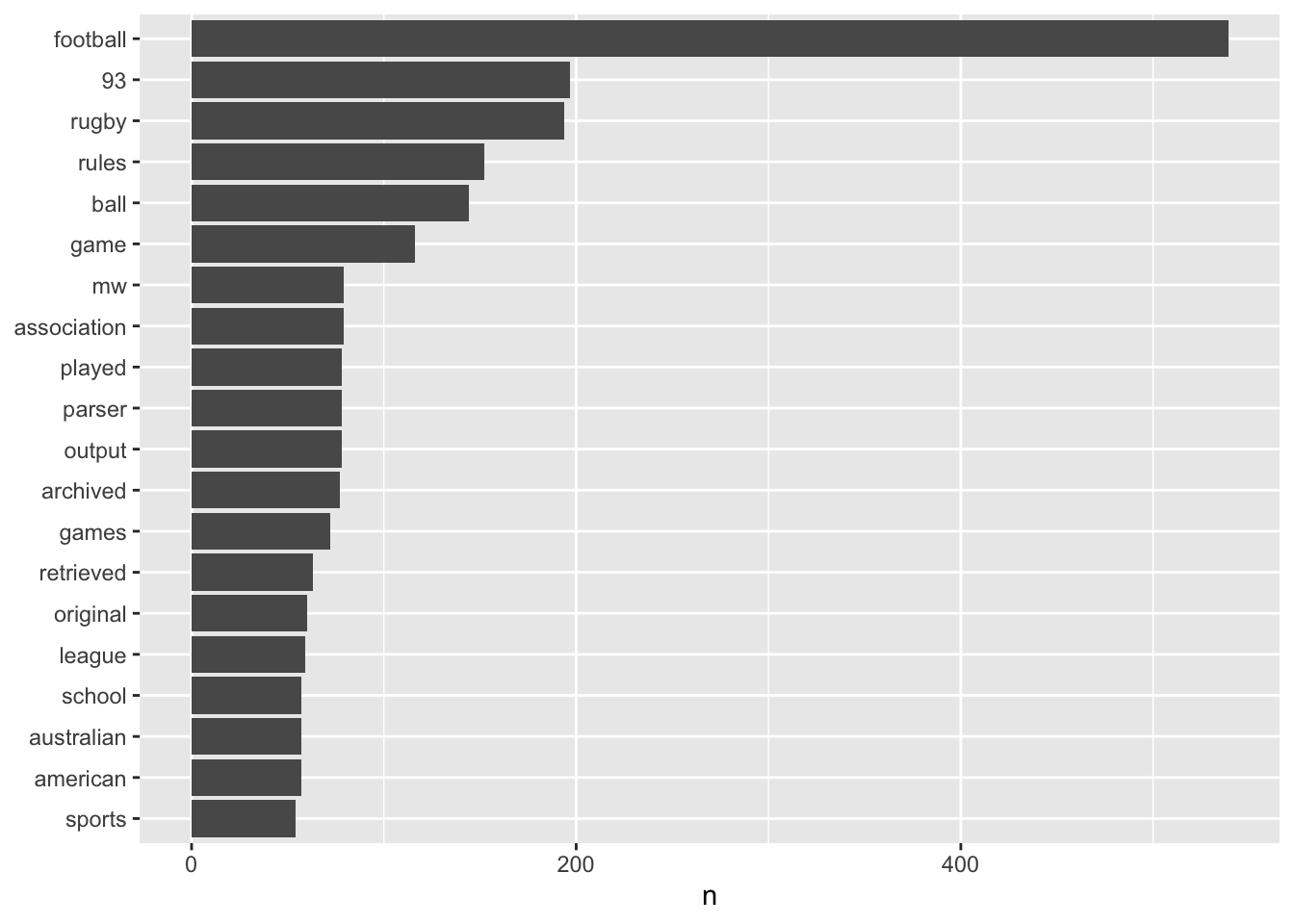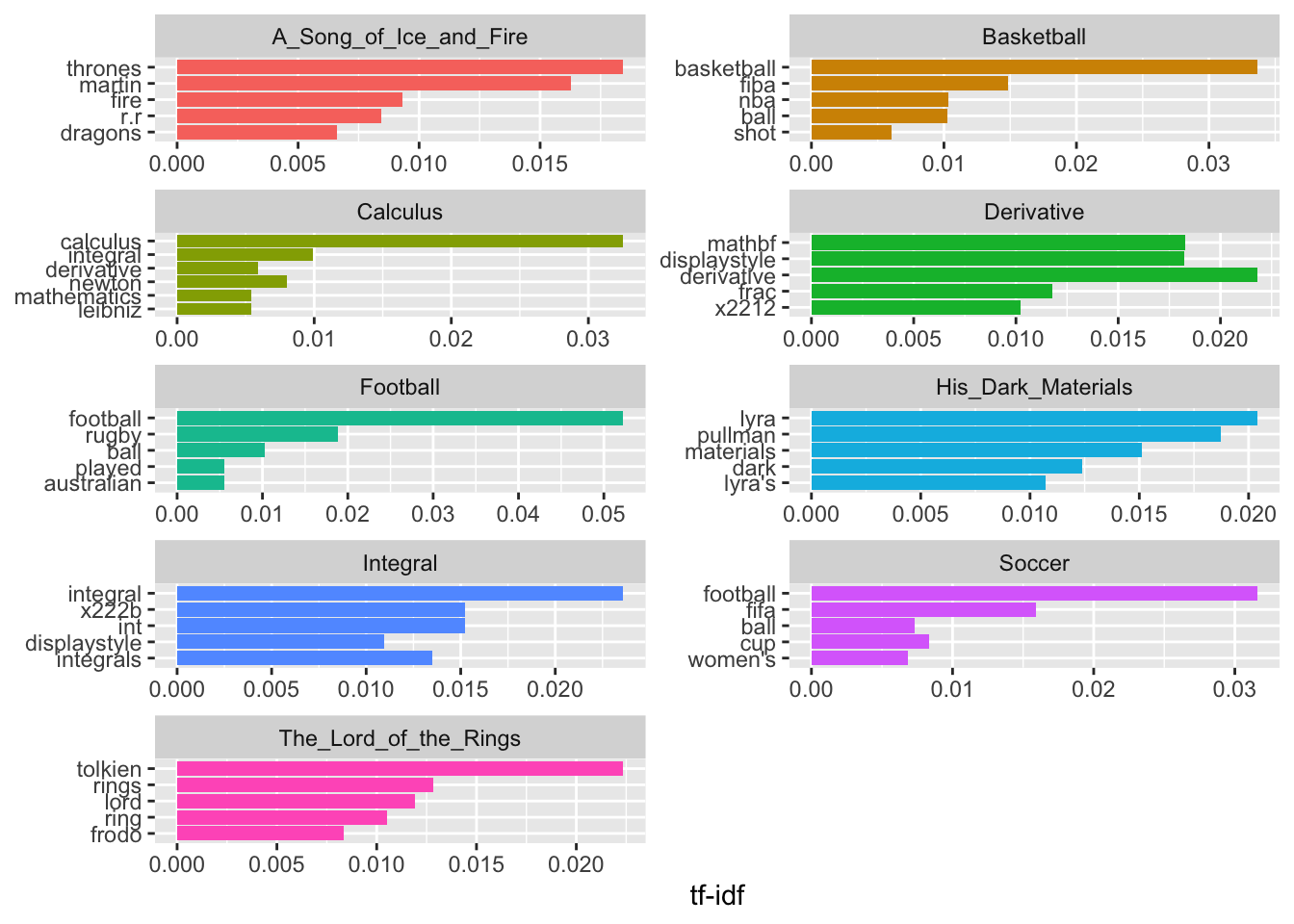# Text mining using TidyText

## Introduction

Text mining is a process of discovering new and latent features within a body of text. It uses Natural Language Processing (NLP) to enable computers to digest human language. It has many uses in the real world. A couple of examples included are:

• Spam Filtering
• Sentiment Analysis
• Fraud Detection
• Knowledge Management

In this post, we will use the package tidytext, which is part of the tidyverse. You can read more about it here. tidytext is a package that uses tidy data principles to wrangle and visualize text data. In this post, we will process some text and maybe discover new information. First, make sure you have the following libraries installed, tidytext, dplyr, ggplot2,stringi, textclean, and forcats.

## Importing the text

#install.packages(c('dplyr','ggplot2','stringi','forcats', 'tidytext','textclean', 'tidyr'))
library(dplyr)
##
## Attaching package: 'dplyr'
## The following objects are masked from 'package:stats':
##
##     filter, lag
## The following objects are masked from 'package:base':
##
##     intersect, setdiff, setequal, union
library(ggplot2)
library(stringi)
library(textclean)
library(tidytext)
library(forcats)
library(tidyr)

We can gather data by mining text from Wikipedia. Let’s import nine articles with topics including math, sports, and fantasy books. The specific topics will be important later when we try to perform text analytics. We will use the function readLines to directly connect to a Wikipedia URL and read all text lines.

wiki <- "http://en.wikipedia.org/wiki/"
titles <- c("Integral", "Derivative",
"A_Song_of_Ice_and_Fire", "The_Lord_of_the_Rings", "His_Dark_Materials")
articles <- character(length(titles))

for (i in 1:length(titles)) {
articles[i] <- stri_flatten(readLines(stri_paste(wiki, titles[i]), warn = F), col = " ")
}
length(articles)
##  9

## Text processing

Now that we have imported our articles, which we will now call documents. We can the documents are represented as a large character vector with a length of 9. For each document, we need to separate each word and then count them. To do so, we use a process called tokenization. While a computer will read strings as a series of character, humans will read it as a sentence of words. To reproduce this for computers, we can split the string using a space delimiter, " ", and then split the sentence into words or in this case, tokens. Let’s take a look at an example. Here we have a random sentence extracted from one of our documents.

text <- c('A Song of Ice and Fire is a series of epic fantasy novels by the American novelist and screenwriter George R. R. Martin. He began the first volume of the series, A Game of Thrones, in 1991, and it was published in 1996.')

We can can use the following code to tokenize this text.

tokenize_text <- (unlist(lapply(text, function (x) strsplit(x, split = ' ' )))) #' '  is used as the space delimiter
tokenize_text
##   "A"            "Song"         "of"           "Ice"          "and"
##   "Fire"         "is"           "a"            "series"       "of"
##  "epic"         "fantasy"      "novels"       "by"           "the"
##  "American"     "novelist"     "and"          "screenwriter" "George"
##  "R."           "R."           "Martin."      "He"           "began"
##  "the"          "first"        "volume"       "of"           "the"
##  "series,"      "A"            "Game"         "of"           "Thrones,"
##  "in"           "1991,"        "and"          "it"           "was"
##  "published"    "in"           "1996."

Fortunately, tokenization is built into the tidytext package. First, we will do some text cleaning by removing some common HTML tags with the function replace_html(). Since HTML tags are not useful information for us, we can discard them. Then we will convert our large character vector into a data frame. We will follow this with unnest_tokens() to tokenize the documents.

articles <-  replace_html(articles, symbol = TRUE)
articles <- data.frame('articles' = articles)
articles$titles <- titles tokenized <- articles %>% unnest_tokens(word, articles) head(tokenized) If you haven’t noticed already, there are still some nonsense words that pertain to HTML code still. We can create a new database with additional HTML code to remove, but for now, this is okay as later we will weight the important words more than the non-important words, which should reduce the importance of the HTML code. Next, let’s count how many times each word appears in each document using the count() function. tokenized %>% count(titles, word, sort = TRUE) %>% head() As you can see, the top words are “the” and “of”, which are not important for understanding the hidden features of the documents. This set of words are called stop words. They are commonly used words in any language, where they are unimportant for NLP, and are removed to focus on the important words. We will load a generic stop_words dataset and filter out these words from our data frame. Please keep in the mind that depending on the context of the documents, you may need a different set of stop words. data(stop_words) tokenized <- tokenized %>% anti_join(stop_words) #this filters out the stop words ## Joining, by = "word" document_words <- tokenized %>% count(titles, word, sort = TRUE) head(document_words) Now we can see important words that make sense for each of our documents, like the word football appearing the most for the Wikipedia article “Football”. Let’s take a look at a list of the most common words in “Football”. document_words[document_words$titles == 'Football',] %>%
filter(n > 50) %>%
mutate(word = reorder(word, n)) %>%
ggplot(aes(n, word)) +
geom_col() +
labs(y = NULL)## Term Frequency and Inverse Document Frequency

Let’s try to contextualize this data by ranking them based on how often they appear within the document, instead of looking at the absolute count. This is called term frequency (TF) and it gives the frequency of the word in each document. The TF is defined as $$tf(t,d)$$: $tf(t,d) = \frac{f_{t,d}}{\Sigma_{k}f_{t,d}}$ where $$f_{t,d}$$ is the raw count of each term. In addition, each document will have its own TF. Let’s calculate it by finding the total number of words that appears per document.

total_words <- document_words %>%
group_by(titles) %>%
summarize(total = sum(n))

document_words <- left_join(document_words, total_words)
## Joining, by = "titles"
head(document_words)

Now we can rank each word by dividing the raw count of the word by the total number of words in the document.

freq_by_rank <- document_words %>%
group_by(titles) %>%
mutate(rank = row_number(),
term frequency = n/total) %>%
ungroup()
head(freq_by_rank)

However, there are also rare words that may be important that may be lost by using TF, since TF is influenced by the length of the document. Here we introduce inverse document frequency (IDF), which weighs the rare words across all documents by dividing the total number of documents by the number of documents containing the term. We add a $+ 1$ term to prevent division by 0. $idf(t,D) = log \frac{N}{|d \in D: t \in d| + 1}$ We can combine this with TF to produce the TF-IDF score. Now TF will be weighted based on if they show up in other documents. $tfidf(t,d,D) = tf(t,d) \times idf(t,D)$

document_tf_idf <- document_words %>%
bind_tf_idf(word, titles, n)
head(document_tf_idf)

We can see that the word 93 in A_Song_of_Ice_and_Fire had a very high term frequency. However, when we weight it to produce the TF_IDF score, it is reduced to a value of 0. Now let’s take a look at the top 5 words per document.

library(forcats)

document_tf_idf %>%
group_by(titles) %>%
slice_max(tf_idf, n = 5) %>%
ungroup() %>%
ggplot(aes(tf_idf, fct_reorder(word, tf_idf), fill = titles)) +
geom_col(show.legend = FALSE) +
facet_wrap(~titles, ncol = 2, scales = "free") +
labs(x = "tf-idf", y = NULL)## Latent Semantic Analysis

For the most part, the top words weighted using TF-IDF makes sense. Now that we have set up our data, we can start digging in deeper to find some relationships between the documents. We will take a look at Latent Semantic Analysis (LSA). It is an NLP technique that analyzes a set of documents and the terms within to find common or divergent concepts related to the documents and terms. We can extend this definition of LSA to be used as a method for classifying documents into different topics. To start, we need to convert our data into a term-document matrix, where the columns corresponds to documents and the rows correspond to terms. Document-term matrix is the transpose version.

tf_idf_matrix <- document_tf_idf %>% select(titles, word, tf_idf)%>% pivot_wider(names_from = titles, values_from = tf_idf, values_fill = 0) %>% as.data.frame()
words <- tf_idf_matrix[]
tf_idf_matrix <- tf_idf_matrix[,-1] #Removing the column containing the words
rownames(tf_idf_matrix) <- words

LSA is a dimensional reduction technique similar to PCA. In fact, to perform it, we calculate the singular value decomposition (SVD) on the document-term matrix. See my other post to learn more about SVD.

$M = U \Sigma V^{T}$ By using LSA, we will reduce our high-dimensional data into lower dimensional data to do one of three things:

• Reduce the data to save on computational work.
• De-noise the data by weighting the more important terms.
• Decrease the sparsity of the matrix by relating synonyms. This means that some dimensions become merged due to related terms. In addition, the effects of words with multiple means are mitigated.

To further reduce the computational load, we will take the top 50 words weighed by TF-IDF from each document.

top_words <- document_tf_idf %>%
group_by(titles) %>%
slice_max(tf_idf, n = 50) %>% pull(word) %>% unique()

We perform LSA by passing our term-document matrix into the functionsvd().

LSA <- svd(tf_idf_matrix[top_words,], 4) #the rank was set to 4
rownames(LSA$u) <- rownames(tf_idf_matrix[top_words,]) rownames(LSA$v) <- colnames(tf_idf_matrix[top_words,])
colnames(LSA$v) <- paste0('LSA_', c(1:dim(LSA$v))) #this renames each of the columns
colnames(LSA$u) <- paste0('LSA_', c(1:dim(LSA$u)))

We take the right-singular vector $$V$$, which contains a set of orthonormal vectors that spans the row space of our term-document matrix. By plotting the first two columns of LSA, we should observe a separation between the documents due to the variance in the first two components.

#install.packages("ggrepel")
library(ggrepel)

df <- LSA$v %>% as.data.frame() %>% select('LSA_1', 'LSA_2') df$title <- rownames(df)

ggplot(df, aes(x = LSA_1, y = LSA_2, color = title )) +
geom_text_repel(aes(label = title), color = 'black') +
coord_fixed()